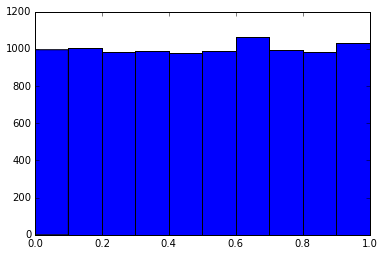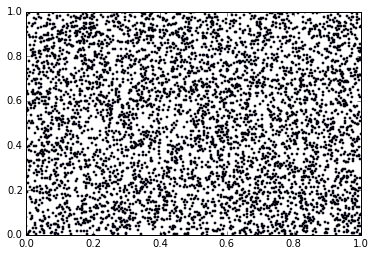## The threefry random number generator

Pseudo-random number generation has many applications in computing. The use of random number in parallel is becoming necessary and a recent solution is the use of so-called block cipher algorithms. In this post, I review the mechanism being the Threefry random number generator that is based on the the Threefish block cipher. The article below is actually a Jupyter notebook and can be downloaded on my GitHub account: see the notebook | download the notebook (a right-click may help). The code is 3-clause BSD and content CC-BY.

As a teasing, Threefry is crush resistant, easy to implement, suitable for parallel applications and a working pure Python code is presented! I was motivated to understand this topic because the nano-dimer program by Peter Colberg uses it. Requirements for a Pseudorandom Number Generator (PRNG) include a good performance and a low memory usage (critical for GPUs). A good overview of these considerations is given in Salmon et al doi:10.1145/2063384.2063405.

## Threefish¶

The Threefish block cipher is a part of the encryption candidate Skein. A block cipher is a reversible transformation of a sequence of bytes that depends on a user chosen key. Threefish (see the Wikipedia page and the official specification) is built of several simple components:

• a mix function that operates on words
• a permutation function $\pi$ that operates on several consecutive words
• a key schedule, that defines how the information in the key is applied within the algorithm

Here, a word is defined as a sequence of 64 bits. In practice, it is stored as an unsigned 64-bit integer. All sums are computed modulo $2^{64}-1$.

The full algorithm, going from a series of "plain text" words $p_i$ to the corresponding "cipher" $c_i$ consists in applying the following steps for $N_r$ rounds:

1. one round in four, add the key to the current value of the data
2. apply the mix function to two consecutive words at a time
3. permute the words according to $\pi$

After the last round, a final addition of the key is applied and the full data is XOR-ed with the initial value $p$. In Threefish, the key schedule is modified by a tweak, an extra set of two words. The specification provides:

• a set of rotation constants for the mix function
• the constant C240 that is part of the key schedule
• the permutation function $\pi$
• the number of rounds.

Threefish is specified for blocks of 256, 512 and 1024 bits. Below is a plain implementation of Threefish-256 that operates on 4 words blocks, in Python (the code is intended for Python 3). The test reproduces the content of the example found on the official NIST CD release of Skein. Intermediate steps are obtained by adding the argument "debug=True" and follow the step-by-step content of the file KAT_MCT/skein_golden_kat_internals.txt.

The code reproduces section 3.3 of the skein specification, with identical or close notation for all symbols, and is hopefully self-explaining.

In :
NW = 4
C240 = 0x1BD11BDAA9FC1A22
N_ROUNDS=72
pi = (0, 3, 2, 1)
R_256 = ((14, 16), (52, 57), (23, 40), (5, 37), (25, 33), (46, 12), (58, 22), (32, 32))

def rotl_64(x, d):
return ((x << d) | (x >> (64-d))) & MASK

def mix(x0, x1, R):
y1 = rotl_64(x1, R) ^ y0
return y0, y1

def key_schedule(K, TW, s):

def threefish(p, K, TW, debug=False):
K = (K, K, K, K, C240^K^K^K^K)
TW = (TW, TW, TW^TW)
v = list(p)
for r in range(N_ROUNDS):
e = *NW
if r%4 == 0:
ksi = key_schedule(K, TW, r//4)
for i in range(NW):
e[i] = (v[i] + ksi[i]) & MASK
if debug: print('key injection   ', list(map(hex, e)))
else:
e = v
f = *NW
f, f = mix(e, e, R_256[r%8])
f, f = mix(e, e, R_256[r%8])
if (r%2 == 0) and debug: print('end of round %03i' % (r+1), list(map(hex, f)))
for i in range(NW):
v[i] = f[pi[i]]
if (r%2 == 1) and debug: print('end of round %03i' % (r+1), list(map(hex, v)))

ksi = key_schedule(K, TW, N_ROUNDS//4)
v = [((x + k)^pp) & MASK for x, k, pp in zip(v, ksi, p)]
if debug: print(list(map(hex, v)))

return v

In :
# Test run with parameters from the NIST CD, file KAT_MCT/skein_golden_kat_internals.txt

K = (0x0,)*NW
TW = (0x0,0x0)

c = threefish((0x0,)*NW, K, TW)
print([hex(x) for x in c])

K = (0x1716151413121110, 0x1F1E1D1C1B1A1918, 0x2726252423222120, 0x2F2E2D2C2B2A2928)
TW = (0x0706050403020100, 0x0F0E0D0C0B0A0908)

c = threefish((0xF8F9FAFBFCFDFEFF, 0xF0F1F2F3F4F5F6F7, 0xE8E9EAEBECEDEEEF, 0xE0E1E2E3E4E5E6E7), K, TW)
print([hex(x) for x in c])

['0x94eeea8b1f2ada84', '0xadf103313eae6670', '0x952419a1f4b16d53', '0xd83f13e63c9f6b11']


## Block ciphers, random numbers and Threefry¶

The motivation for this post (and the corresponding code) is to generate random numbers. The use of cryptographic protocols as keyed counter-based pseudorandom number generators is presented in Salmon et al "Parallel Random Numbers: As Easy as 1, 2, 3", doi:10.1145/2063384.2063405, Proceedings of SC'11. The use of the the Skein algorithm (based on Threefish) to generate random numbers is already mentioned in its specification, but the paper by Salmon et al goes further by specializing the algorithm (and others as well) for use in a PRNG context. I present here their specialization of Threefish: Threefry. Threefry is based on the Threefish block cipher, with three shortcuts. The number of rounds is reduced, the tweak that influence the key schedule is removed and the final XOR-ing of the output with the input data is removed. This latter shortcut is not explicit in the manuscript, as far as I understand. I concentrate here on Threefry-2x64, that is using two 64-bit words, with 20 rounds.

Whereas the prototypical PRNG steps from integer value to integer value using (more or less) complex iteration schemes, a counter based PRNG gives a random value directly for any point in the sequence at a fixed computational cost. The purpose of a cryptographic algorithm is to make it hard to guess the input from the output. Changing the input value from $i$ to $i+1$ will output an uncorrelated value.

The algorithm depends on a key of two words. One of them can be used as the seed and the other to identify one stream (i.e. a compute unit in a parallel computing environment). The position in the random stream is given as the "plain text" word.

Practically, it means that instead of keeping a state for the PRNG it is possible to request random_number(counter, key) where random_number is a pure function, counter is simply the iteration number that is requested and key is used to identify the stream (including a stream "id" and a seed).

The authors of the paper provide an implementation at http://www.thesalmons.org/john/random123/ or http://www.deshawresearch.com/resources_random123.html

As I wanted to understand the algorithm and have a working Threefish above, here is a Python version of Threefry-2x64.

In :
NW_THREEFRY = 2
C240 = 0x1BD11BDAA9FC1A22
N_ROUNDS_THREEFRY = 20
R_THREEFRY = (16, 42, 12, 31, 16, 32, 24, 21)

def key_schedule_threefry(K, s):

def threefry(p, K, debug=False):
K = (K, K, C240^K^K)
v = list(p)
for r in range(N_ROUNDS_THREEFRY):
if r%4 == 0:
ksi = key_schedule_threefry(K, r//4)
if debug: print('key injection   ', list(map(hex, e)))
else:
e = v
v, v = mix(e, e, R_THREEFRY[r%8])
if debug: print('end of round %03i' % (r+1), list(map(hex, v)))

ksi = key_schedule_threefry(K, N_ROUNDS_THREEFRY//4)
v = [(x + k) & MASK for x, k in zip(v, ksi)]
if debug: print(list(map(hex, v)))

return v


Test output from the Random123 distribution, in the file Random123-1.08/examples/kat_vectors.

In :
print(list(map(hex, threefry((0x0,0x0), (0x0, 0x0)))))
ff = 0xffffffffffffffff
print(list(map(hex, threefry((ff,ff), (ff, ff)))))
print(list(map(hex, threefry((0x243f6a8885a308d3, 0x13198a2e03707344), (0xa4093822299f31d0, 0x082efa98ec4e6c89)))))

['0xc2b6e3a8c2c69865', '0x6f81ed42f350084d']
['0xe02cb7c4d95d277a', '0xd06633d0893b8b68']
['0x263c7d30bb0f0af1', '0x56be8361d3311526']


## Using Threefry for random numbers¶

Below, illustrate the use of Threefry as one would use a typical PRNG. A seed is chosen, from which the key K is built. Then a loop uses the threefry function to generate two 64-bit words. The data is collected and show in a histogram and a next-point correlation plot. Also, the random numbers are used to estimate $\pi$ via the Monte Carlo method.

In :
SEED = 0x1234
K = (0x0, SEED)
MULT = 1/2**64
data = []
total_count = 10000
pi_count = 0
for i in range(total_count):
c0, c1 = threefry((i, 0x0), K)
c0 *= MULT
c1 *= MULT
data.append(c0)
if c0**2+c1**2<1:
pi_count += 1

print("Estimate of pi", 4*pi_count/total_count)

Estimate of pi 3.1228

In :
import numpy as np
%matplotlib inline
import matplotlib.pyplot as plt

In :
data = np.array(data)
plt.hist(data);In :
plt.scatter(data[0::2], data[1::2], s=3); plt.xlim(0,1); plt.ylim(0,1);